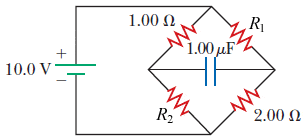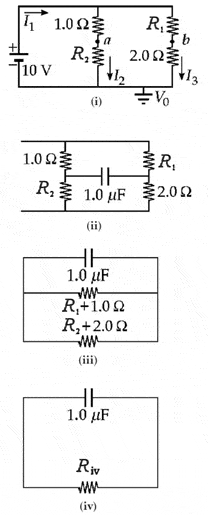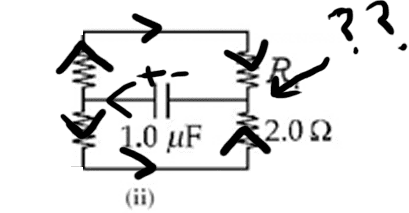# Discharging capacitor in RC circuit, confusing path

## Homework Statement

The circuit in the figure below has been connected for a long time. Let R1 = 8.20 Ω and R2 = 4.40 Ω.(a) What is the potential difference across the capacitor?
(b) If the battery is disconnected from the circuit, over what time interval does the capacitor discharge to one-eighth its initial voltage?

## Homework Equations

V=IR
Q=CV
Derived equations for current/voltage over time in discharging case

## The Attempt at a Solution

(a) wasn't hard. I think normally, if this capacitor was fully in series with the resistors, then the potential difference across the capacitor would be equal to the voltage across the battery. However, in this case, it's not in series. Once the capacitor becomes fully charged, the current in the middle branch stops completely and the capacitor acts as an infinite resistor, but it keeps going everywhere else. Then the 1.00 Ω resistor and R2 are in series. I can use that to solve for the current across that left branch containing those two resistors while the capacitor is fully charged, because parallel devices have equal voltage drops, so I can use V=IR (or 10.0 = I*[1.00Ω + R2]), and then do so similarly for the right branch to find the other current. Then I can use Kirchoff's loop rule in that upper triangle to solve for the difference of potential across the capacitor.

(b) doesn't make a lot of sense to me. First, which way would the resulting current be? To the left because of the fact that there's less resistance coming from that direction so the + would be on the left of the capacitor?

The part that I understand the least, though, is that apparently the Reff actually becomes a combination of all of the resistors. Somehow they can be combined like this. This first and second pictures make perfect sense, but I don't understand the rest.Homework Helper
Gold Member
2020 Award
When the capacitor discharges with the battery disconnected, the circuit is quite simple: R1 is in series with the 1 ohm resistor, and R2 is in series with the 2 ohm resistor. The two series chains are in parallel so the total resistance can easily be computed. One other hint for this part: Can you write the equation of the voltage across the capacitor and/or the current as the capacitor discharges?

When the capacitor discharges with the battery disconnected, the circuit is quite simple: R1 is in series with the 1 ohm resistor, and R2 is in series with the 2 ohm resistor. The two series chains are in parallel so the total resistance can easily be computed. One other hint for this part: Can you write the equation of the voltage across the capacitor and/or the current as the capacitor discharges?
I guess I can somewhat see how it's in series, but this doesn't make much sense to me.This is basically an equivalent circuit after the voltage source is removed, but I can't make sense of this. They're in series, but what happens to the current? Does it go back towards the capacitor?

NascentOxygen
Staff Emeritus